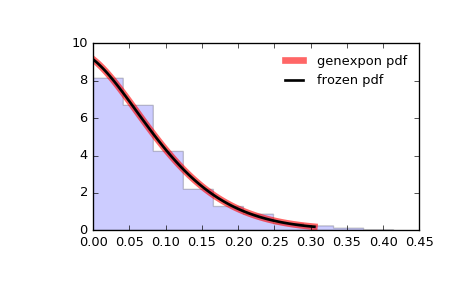# scipy.stats.genexpon¶

scipy.stats.genexpon = <scipy.stats._continuous_distns.genexpon_gen object at 0x2b2318c1d6d0>[source]

A generalized exponential continuous random variable.

As an instance of the rv_continuous class, genexpon object inherits from it a collection of generic methods (see below for the full list), and completes them with details specific for this particular distribution.

Notes

The probability density function for genexpon is:

genexpon.pdf(x, a, b, c) = (a + b * (1 - exp(-c*x))) *                                    exp(-a*x - b*x + b/c * (1-exp(-c*x)))


for x >= 0, a, b, c > 0.

genexpon takes a, b and c as shape parameters.

The probability density above is defined in the “standardized” form. To shift and/or scale the distribution use the loc and scale parameters. Specifically, genexpon.pdf(x, a, b, c, loc, scale) is identically equivalent to genexpon.pdf(y, a, b, c) / scale with y = (x - loc) / scale.

References

H.K. Ryu, “An Extension of Marshall and Olkin’s Bivariate Exponential Distribution”, Journal of the American Statistical Association, 1993.

N. Balakrishnan, “The Exponential Distribution: Theory, Methods and Applications”, Asit P. Basu.

Examples

>>> from scipy.stats import genexpon
>>> import matplotlib.pyplot as plt
>>> fig, ax = plt.subplots(1, 1)


Calculate a few first moments:

>>> a, b, c = 9.13, 16.2, 3.28
>>> mean, var, skew, kurt = genexpon.stats(a, b, c, moments='mvsk')


Display the probability density function (pdf):

>>> x = np.linspace(genexpon.ppf(0.01, a, b, c),
...                 genexpon.ppf(0.99, a, b, c), 100)
>>> ax.plot(x, genexpon.pdf(x, a, b, c),
...        'r-', lw=5, alpha=0.6, label='genexpon pdf')


Alternatively, the distribution object can be called (as a function) to fix the shape, location and scale parameters. This returns a “frozen” RV object holding the given parameters fixed.

Freeze the distribution and display the frozen pdf:

>>> rv = genexpon(a, b, c)
>>> ax.plot(x, rv.pdf(x), 'k-', lw=2, label='frozen pdf')


Check accuracy of cdf and ppf:

>>> vals = genexpon.ppf([0.001, 0.5, 0.999], a, b, c)
>>> np.allclose([0.001, 0.5, 0.999], genexpon.cdf(vals, a, b, c))
True


Generate random numbers:

>>> r = genexpon.rvs(a, b, c, size=1000)


And compare the histogram:

>>> ax.hist(r, normed=True, histtype='stepfilled', alpha=0.2)
>>> ax.legend(loc='best', frameon=False)
>>> plt.show()Methods

 rvs(a, b, c, loc=0, scale=1, size=1, random_state=None) Random variates. pdf(x, a, b, c, loc=0, scale=1) Probability density function. logpdf(x, a, b, c, loc=0, scale=1) Log of the probability density function. cdf(x, a, b, c, loc=0, scale=1) Cumulative distribution function. logcdf(x, a, b, c, loc=0, scale=1) Log of the cumulative distribution function. sf(x, a, b, c, loc=0, scale=1) Survival function (also defined as 1 - cdf, but sf is sometimes more accurate). logsf(x, a, b, c, loc=0, scale=1) Log of the survival function. ppf(q, a, b, c, loc=0, scale=1) Percent point function (inverse of cdf — percentiles). isf(q, a, b, c, loc=0, scale=1) Inverse survival function (inverse of sf). moment(n, a, b, c, loc=0, scale=1) Non-central moment of order n stats(a, b, c, loc=0, scale=1, moments='mv') Mean(‘m’), variance(‘v’), skew(‘s’), and/or kurtosis(‘k’). entropy(a, b, c, loc=0, scale=1) (Differential) entropy of the RV. fit(data, a, b, c, loc=0, scale=1) Parameter estimates for generic data. expect(func, args=(a, b, c), loc=0, scale=1, lb=None, ub=None, conditional=False, **kwds) Expected value of a function (of one argument) with respect to the distribution. median(a, b, c, loc=0, scale=1) Median of the distribution. mean(a, b, c, loc=0, scale=1) Mean of the distribution. var(a, b, c, loc=0, scale=1) Variance of the distribution. std(a, b, c, loc=0, scale=1) Standard deviation of the distribution. interval(alpha, a, b, c, loc=0, scale=1) Endpoints of the range that contains alpha percent of the distribution

#### Previous topic

scipy.stats.genpareto

#### Next topic

scipy.stats.genextreme